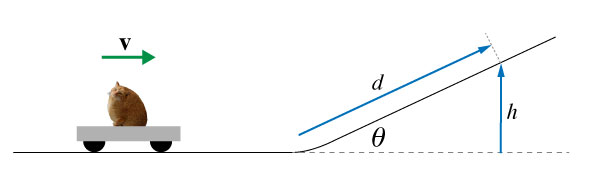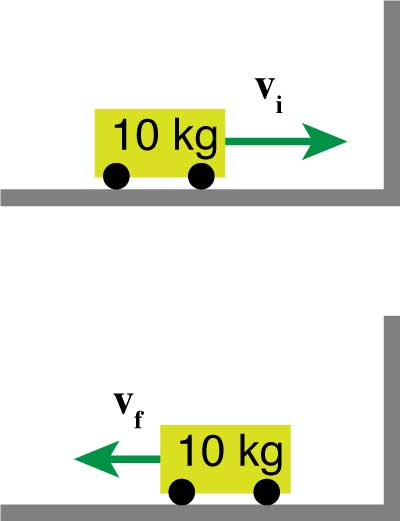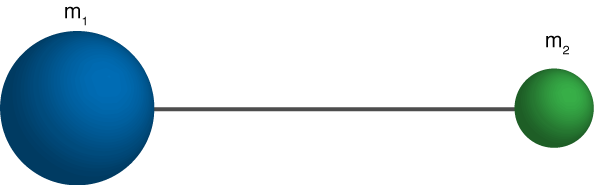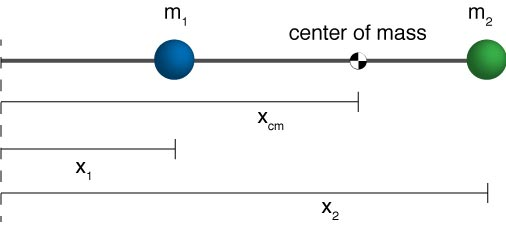Mr Mittens is moving towards a frictionless ramp at a constant velocity...# Introduction

We’ve assumed that forces acted continuously.

But, really they have durations in time.

For collisions, we have tools to analyze how these interactions work.

How long is a golf club in contact with a golf ball for?

#### Analyze the club-ball collision## ImpulseWe’ll call the area under this curve impulse, $J$.

$$J = F_\textrm{avg} \Delta t$$

It tells us:

a) for how long the force was acting and,
b) how strong the force was during that time.

#### Let's make life a little easierThe area under this triangle is easy to figure out.

However, in the case a non uniform force, we can rely on integration techniques.

$$\mathbf{J} = \int_{t_i}^{t_f} F(t) dt$$

#### Let's make life a little easierThe area under this triangle is easy to figure out.

## Linear Momentum

The linear momentum , $\overrightarrow{p}$, of a particle or an object of mass m moving with a velocity $v$ is defined to be the product of the mass and velocity.

$$\bbox[10px ,border:3px solid red]{\overrightarrow{p} = m \overrightarrow{v}}$$
1. Linear momentum is a vector quantity
2. Its direction is the same as the direction of $\overrightarrow{v}$
3. The dimensions are ML / T
4. SI units are kg · m / s

#### Some example momentums

Momentum of a mosquito:
$m_\textrm{mosquito} = 2.5 \times 10^{−6}$ kg
$v_\textrm{mosquito} = .3$ m/s
$p_\textrm{mosquito} = m \cdot v = 7.5 \times 10^{-7}$ kg ∙ m/s

Momentum of a truck:
$m_\textrm{truck} = 10,000$ kg
$v_\textrm{truck} = 100$ km/hr = 28 m/s
$p_\textrm{truck} = m \cdot v = 2.8 \times 10^5$ kg ∙ m/s

What’s $\Delta \overrightarrow{p}$ of the cart after bouncing off the wall? ($+x$ is to the right)## Impulse-momentum theorem

The impulse delivered to an object equals the object’s change in momentum.

$$\overrightarrow{J} = \overrightarrow{p}_f -\overrightarrow{p}_i = \Delta \overrightarrow{p}$$

Or, we can always rearrange this equation a little bit:

$$\overrightarrow{p}_f = \overrightarrow{p}_i + J$$We can use Newton's second law to make a little more sense out of this:

$$\sum \mathbf{F} = m\mathbf{a} = m \frac{\Delta v}{\Delta t}$$
$$\mathbf{F} \Delta t = m\mathbf{v}_f - m\mathbf{v}_i = \Delta \mathbf{p}$$

Thus we can say that the impulse on an object is equal to its change in momentum

$$\bbox[10px ,border:3px solid red]{\mathbf{J} = \Delta \mathbf{p}}$$

#### Re-write the second law:

“If no net external forces act on a particle, then the particle’s momentum is constant”

$$\sum \mathbf{F} = 0 \Rightarrow \frac{\Delta \mathbf{p}}{\Delta t} = 0$$

Also: the concept of inertia

#### Re-write the second law:

“If no net external forces act on a particle, then the particle’s momentum is constant”

$$\sum \mathbf{F} = 0 \Rightarrow \frac{d \mathbf{p}}{d t} = 0$$

Also: the concept of inertia

A 1 lb bouncy ball and a 1 lb ball of clay are thrown at a wall with equal speeds. The bouncy ball bounces, the clay sticks. Which object exerts a larger impulse on the wall?

1. They exert equal impulses because they have equal momenta.
2. The clay ball exerts a larger impulse because it sticks.
3. Neither exerts an impulse on the wall because the wall doesn’t move.
4. The rubber ball exerts a larger impulse because it bounces.

A conservation law states that some quantity of an isolated system never changes as the system evolves in time.

1. "some quantity" : this could be things like number of particles, amount of energy.
2. "isolated system" : the universe is really the only isolated system. Everything else is only approximately isolated.
3. "evolves in time": this is just how the systems changes with respect to time.

## Conservation of Momentum

Total momentum before: $$p = mv_1 - mv_2$$Total momentum after $$p = -mv_1 + mv_2$$#### Why is momentum conserved?Noether's Theorem

A 3 kg cat sitting on a 1.5 kg piece of cardboard on a frozen lake wants to jump to shore without touching the ice. If there is no friction between the cardboard and the ice, when the cat jumps horizontally to the shore. the cardboard will move in the opposite direction with a velocity:

1. half as great as the cat's velocity.
2. equal to the cat's velocity.
3. twice as great as the cat's velocity.
4. four times as great as the cat's velocity.

Two people are standing on opposite ends of a very large (≈ 4 meter long) skateboard. The skateboard is initially at rest with respect to the ground. The person on the right throws a very massive ball to the left, and the person on the left catches it. After the ball is caught (ignoring friction between the road and the skateboard), the skateboard is:

1. stationary.
2. moving to the right.
3. moving to the left.
4. can't say because we would need to know the masses, the velocities, etc.

A 5000 kg train car is moving at 22.0 m/s. It starts raining, a lot. Later, the car is rolling at a speed of 20 m/s. Assuming the only thing that changed was the mass of the car due to the water, how much water has been collected in the car?

## Types of Collisions

Elastic: An elastic collision involves no loss of energy. That is, the kinetic energy is the same before and after.

Inelastic: During an inelastic collision, some of the kinetic energy of the kinetic might be converted to heat energy and thus the total KE might be different before and after. **If the two object stick together after the collision, then the collision is called 'completely inelastic'. **

#### 1-D elastic collision

Let's have an elastic collision between these two marbles.

Marble 1 has a mass of .25 kg. Marble 2 has a mass of .80 kg. Marble 2 moves at 5 meters per second and strikes marble 2 which is at rest. What is the motion that follows the collision?

blank

A bird that has a mass of 300 grams is flying at 6 m/s. A little insect with mass 10 grams is flying directly towards the bird. The bird opens his mouth and the insect gets eaten. If the insect was traveling at 13 m/s, what is the speed of the bird post-lunch.

## In class: The ballistic pendulumBased on the angle $\theta$, we can figure out how fast the bullet was traveling.

• Mass of bullet: $m_B$ = 0.068 kg
• Mass of block: $m_W$ = 260 g
• Height of block after collision: $h_f$ = To Measure!
• Initial velocity of the block: $v_{02}$ = 0 m/s
• Initial velocity of the bullet: $v_{01}$ = Unknown

A hockey puck is sliding on ice at 12 m/s. It is hit with a hockey stick which delivers an impulse of -4.0 kg m /s. This causes the puck to travel in the opposite direction at the same speed. What is the mass of the puck?

## Two-dimensional collisions

Here is a collision between 3 marbles taking place in 2 dimensions.

Momentum is conserved!

However, we have to remember that momentum is a vector.
Thus, in 2D, the $x$ momentum is conserved, and the $y$ momentum is conserved. We'll need to separate these components.

One ball of clay is traveling in the +x direction at 3.0 m/s. It collides with another ball of clay traveling in the +y direction at 2.0 m/s. Ball 1 has a mass of 20 g while ball 2 has a mass of 30g. What is the speed and direction of the resulting 50 g ball of clay?

A hockey puck is sliding on ice at 12 m/s. It is hit with a hockey stick which delivers an impulse of 4.0 kg m /s. This causes the puck to travel in the opposite direction at the same speed. What is the mass of the puck?

A 10 gram particle is traveling east at 2.0 m/s when it explodes into 3 pieces. A 3 gram fragment goes west at 10 m/s while another 3 gram fragment travels 40 degrees north of east at 12m/s. What are the speed and direction of the third fragment?Gravity is a force acting on this blue pole. It acts everywhere, but if we needed to pick a place, $r$, to put the force arrow, where should we put it?

What is $r$?## Center of Mass

There is a special point in a system or object, called the center of mass, (CM) that moves as if all of the mass of the system is concentrated at that point

The system will move as if an external force were applied to the total mass M located at the center of mass.

For a simple case, like two equal masses on a stick, our intuition should tell us that the center of mass is just in the center of the rod.But, intuition is not enough sometimes...Center of mass of a system like the following will be given by:$$x_{cm} = \frac{m_1 x_1 + m_2 x_2}{m_1+m_2}$$

For a system of $n$ particles, we can generalize into the following. If:

$$\mathbf{r} = x \mathbf{\hat{i}} + y \mathbf{\hat{j}} + z \mathbf{\hat{k}}$$

then,

$$\mathbf{r}_{CM} = \frac{1}{M} \sum_{i=1}^n m_i \mathbf{r}_i$$

Center of mass of a system like the following will be given by:$$x_{cm} = \frac{m_1 x_1 + m_2 x_2}{m_1+m_2}$$

There are 3 object located on the x axis: Their masses are 1,2, and 3 kg and positions are x = 1m, 2m, and 3m, respectively. Draw this system and locate the center of mass

Find the center of mass of these three particles: (assume all masses are the same)#### Newton's laws for a system of particles.

$$\overrightarrow{F}_\textrm{net} = M \overrightarrow{a}_\textrm{com}$$
1. $\overrightarrow{F}_\textrm{net}$ is the net force of all external forces. (No internal forces)
2. $M$ is the total mass of the system.
3. $\overrightarrow{a}_\textrm{com}$ is the acceleration of the center of mass.

#### Solid Bodies

$$\begin{equation} x_\textrm{com} = \frac{1}{M} \int x \; dm \end{equation}$$ $$\begin{equation} y_\textrm{com} = \frac{1}{M} \int y \; dm \end{equation}$$ $$\begin{equation} z_\textrm{com} = \frac{1}{M} \int z \; dm \end{equation}$$

Find the center of mass of a uniform rod of length L and mass M.

Find the center of mass of this triangle.